Thursday, August 5, 2021
Home > ICSE Solutions for Class 10 Maths > S Chand ICSE Maths Solutions Class 10 Chapter 5 Quadratic Equations Exercise 5C

# S Chand ICSE Maths Solutions Class 10 Chapter 5 Quadratic Equations Exercise 5C# S Chand ICSE Maths Solutions Class 10 Chapter 5 Quadratic Equations Exercise 5C

Hi students, Welcome to Amans Maths Blogs (AMB). In this post, you will get S Chand ICSE Maths Solutions Class 10 Chapter 3 Quadratic Equations Exercise 5C. This is the chapter of introduction of ‘Quadratic Equations‘ included in ICSE 2021 Class 10 Maths syllabus.

## S Chand ICSE Maths Solutions Class 10 Chapter 5 Quadratic Equations Exercise 5C

S Chand ICSE Maths Solutions Class 10 Quadratic Equations Exercise 5C: Ques No 1

2x2 + x – 3 = 0

S Chand ICSE Maths Solutions:

Given that 2x2 + x – 3 = 0.

Then, comparing this quadratic equation with ax2 + bx + c = 0, we get a = 2, b = 1, c = -3

Putting the values of a, b and c quadratic formula x = [- b ± √(b2 – 4ac)]/2a.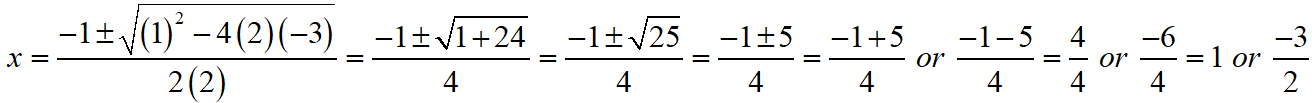Thus, the roos of the given equation are x = 1 or -3/2.

S Chand ICSE Maths Solutions Class 10 Quadratic Equations Exercise 5C: Ques No 2

6x2 + 7x – 20 = 0

S Chand ICSE Maths Solutions:

Given that 6x2 + 7x – 20 = 0.

Then, comparing this quadratic equation with ax2 + bx + c = 0, we get a = 6, b = 7, c = -20

Putting the values of a, b and c quadratic formula x = [- b ± √(b2 – 4ac)]/2a.Thus, the roos of the given equation are x = 4/3 or -5/2.

S Chand ICSE Maths Solutions Class 10 Quadratic Equations Exercise 5C: Ques No 3

9x2 + 6x = 35

S Chand ICSE Maths Solutions:

Given that 9x2 + 6x = 35 ⇒ 9x2 + 6x – 35 = 0.

Then, comparing this quadratic equation with ax2 + bx + c = 0, we get a = 9, b = 6, c = -35

Putting the values of a, b and c quadratic formula x = [- b ± √(b2 – 4ac)]/2a.Thus, the roos of the given equation are x = 5/3 or -7/3.

S Chand ICSE Maths Solutions Class 10 Quadratic Equations Exercise 5C: Ques No 4

3x2 + 7x – 6 = 0

S Chand ICSE Maths Solutions:

Given that 3x2 + 7x – 6 = 0.

Then, comparing this quadratic equation with ax2 + bx + c = 0, we get a = 3, b = 7, c = -6

Putting the values of a, b and c quadratic formula x = [- b ± √(b2 – 4ac)]/2a.Thus, the roos of the given equation are x = 2/3 or -3.

S Chand ICSE Maths Solutions Class 10 Quadratic Equations Exercise 5C: Ques No 5

x2 – 66x + 189 = 0

S Chand ICSE Maths Solutions:

Given that x2 – 66x + 189 = 0.

Then, comparing this quadratic equation with ax2 + bx + c = 0, we get a = 1, b = -66, c = 189

Putting the values of a, b and c quadratic formula x = [- b ± √(b2 – 4ac)]/2a.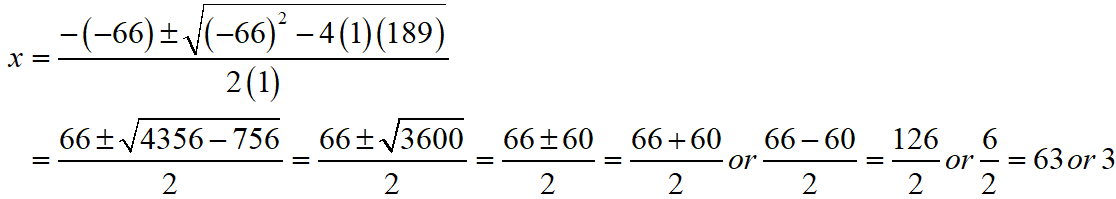Thus, the roos of the given equation are x = 63 or 3.

S Chand ICSE Maths Solutions Class 10 Quadratic Equations Exercise 5C: Ques No 6

√3x2 + 11x + 6√3 = 0

S Chand ICSE Maths Solutions:

Given that √3x2 + 11x + 6√3 = 0.

Then, comparing this quadratic equation with ax2 + bx + c = 0, we get a = √3, b = 11, c = 6√3

Putting the values of a, b and c quadratic formula x = [- b ± √(b2 – 4ac)]/2a.Thus, the roos of the given equation are x = -2√3/3 or -3√3.

S Chand ICSE Maths Solutions Class 10 Quadratic Equations Exercise 5C: Ques No 7

36x2 + 23 = 60x

S Chand ICSE Maths Solutions:

Given that 36x2 + 23 = 60x ⇒ 36x2 – 60x + 23 = 0.

Then, comparing this quadratic equation with ax2 + bx + c = 0, we get a = 36, b = -60, c = 23

Putting the values of a, b and c quadratic formula x = [- b ± √(b2 – 4ac)]/2a.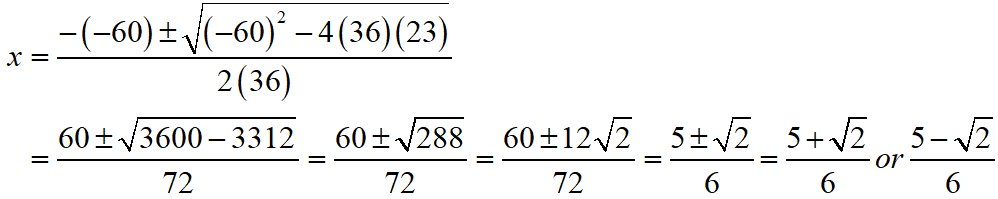Thus, the roos of the given equation are x = (5 + √2)/6 or (5 – √2)/6.

S Chand ICSE Maths Solutions Class 10 Quadratic Equations Exercise 5C: Ques No 8

x2 – 2x + 5 = 0

S Chand ICSE Maths Solutions:

Given that x2 – 2x + 5 = 0.

Then, comparing this quadratic equation with ax2 + bx + c = 0, we get a = 1, b = -2, c = 5

Putting the values of a, b and c quadratic formula x = [- b ± √(b2 – 4ac)]/2a.Thus, the roos of the given equation are x = (1 + 2√(-1)) or (1 – 2√(-1)).

S Chand ICSE Maths Solutions Class 10 Quadratic Equations Exercise 5C: Ques No 9

3x2 – 17x + 25 = 0

S Chand ICSE Maths Solutions:

Given that 3x2 – 17x + 25 = 0.

Then, comparing this quadratic equation with ax2 + bx + c = 0, we get a = 3, b = -17, c = 25

Putting the values of a, b and c quadratic formula x = [- b ± √(b2 – 4ac)]/2a.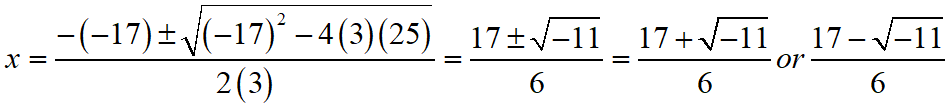Thus, the roos of the given equation are x = (17 + √(-11))/6 or (17 – √(-11))/6.

S Chand ICSE Solutions Class 10 Maths Quadratic Equations Exercise 5C: Ques No 10

15x2 – 28 = x

S Chand ICSE Maths Solutions:

Given that 15x2 – 28 = x ⇒ 15x2 – x – 28 = 0.

Then, comparing this quadratic equation with ax2 + bx + c = 0, we get a = 15, b = -1, c = -28

Putting the values of a, b and c quadratic formula x = [- b ± √(b2 – 4ac)]/2a.Thus, the roos of the given equation are x = 7/5 or -4/3.

S Chand ICSE Maths Solutions Class 10 Quadratic Equations Exercise 5C: Ques No 11

x2 + 3x – 3 = 0, giving your answer correct to two decimal places.

S Chand ICSE Maths Solutions:

Given that x2 + 3x – 3 = 0.

Then, comparing this quadratic equation with ax2 + bx + c = 0, we get a = 1, b = 3, c = -3

Putting the values of a, b and c quadratic formula x = [- b ± √(b2 – 4ac)]/2a.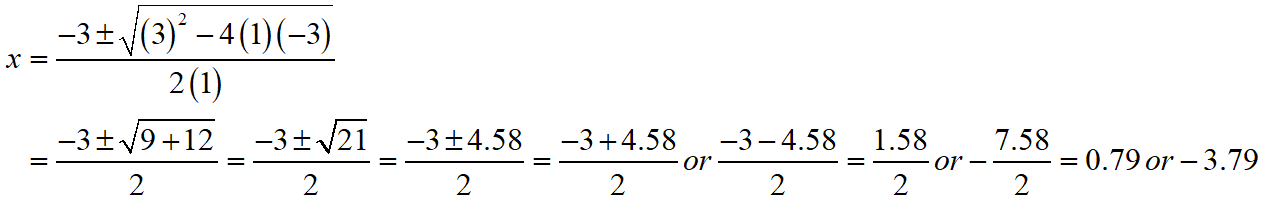Thus, the roos of the given equation are x = 0.79 or -3.79.

S Chand ICSE Maths Solutions Class 10 Quadratic Equations Exercise 5C: Ques No 12

(2/3)x = (-1/6)x2 – 1/3, giving your answer correct to two decimal places.

S Chand ICSE Maths Solutions:

Given that (2/3)x = (-1/6)x2 – 1/3 ⇒ x2 + 4x + 2 = 0.

Then, comparing this quadratic equation with ax2 + bx + c = 0, we get a = 1, b = 4, c = 2

Putting the values of a, b and c quadratic formula x = [- b ± √(b2 – 4ac)]/2a.Thus, the roos of the given equation are x = 3.41 or -0.59.

S Chand ICSE Maths Solutions Class 10 Quadratic Equations Exercise 5C: Ques No 13

x2 + 6x – 10 = 0

S Chand ICSE Maths Solutions:

Given that x2 + 6x – 10 = 0.

Then, comparing this quadratic equation with ax2 + bx + c = 0, we get a = 1, b = 6, c = -10

Putting the values of a, b and c quadratic formula x = [- b ± √(b2 – 4ac)]/2a.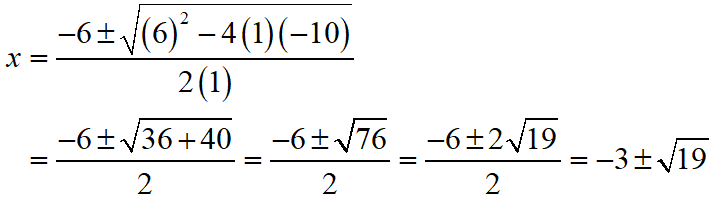Thus, the roos of the given equation are x = -3-√19 or -3+√19.

S Chand ICSE Solutions Class 10 Math Quadratic Equations Exercise 5C: Ques No 14

(x2 + 8)/11 = 5x – x2 – 5

S Chand ICSE Maths Solutions:

Given that (x2 + 8)/11 = 5x – x2 – 5 ⇒ 12x2 – 55x + 63 = 0.

Then, comparing this quadratic equation with ax2 + bx + c = 0, we get a = 12, b = -55, c = 63

Putting the values of a, b and c quadratic formula x = [- b ± √(b2 – 4ac)]/2a.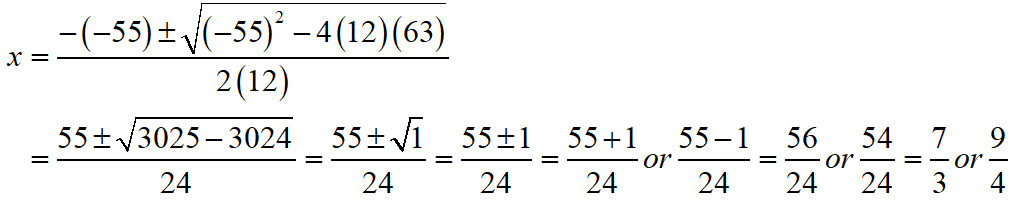Thus, the roos of the given equation are x = 7/3 or 9/4.

S Chand ICSE Solutions Class 10 Math Quadratic Equations Exercise 5C: Ques No 15

y – 3/y = 1/2

S Chand ICSE Maths Solutions:

Given that y – 3/y = 1/2 ⇒ 2y2 – y – 6 = 0.

Then, comparing this quadratic equation with ay2 + by + c = 0, we get a = 2, b = -1, c = -6

Putting the values of a, b and c quadratic formula y = [- b ± √(b2 – 4ac)]/2a.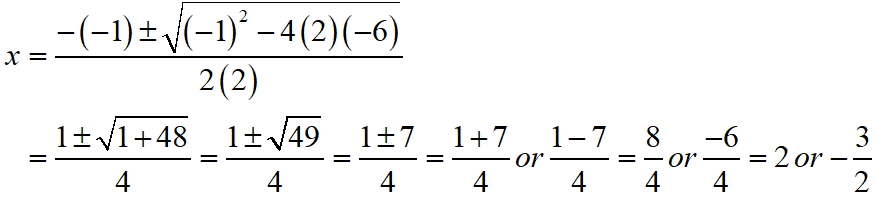Thus, the roos of the given equation are y = 2 or -3/2.

S Chand ICSE Solutions Class 10 Math Quadratic Equations Exercise 5C: Ques No 16

2x + 4/x = 9

S Chand ICSE Maths Solutions:

Given that 2x + 4/x = 9 ⇒ 2x2 – 9x + 4 = 0.

Then, comparing this quadratic equation with ax2 + bx + c = 0, we get a = 2, b = -9, c = 4

Putting the values of a, b and c quadratic formula x = [- b ± √(b2 – 4ac)]/2a.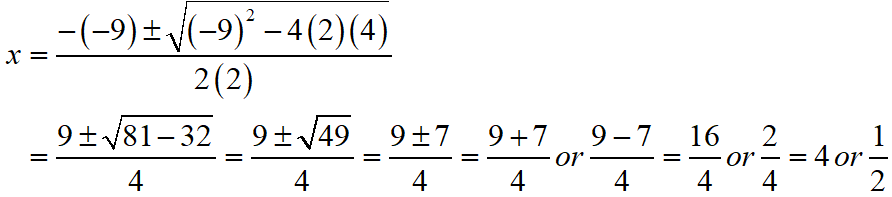Thus, the roos of the given equation are x = 4 or 1/2.

S Chand ICSE Solutions Class 10 Math Quadratic Equations Exercise 5C: Ques No 17

x/(x + 1) + (x + 1)/x = 34/15, x ≠ 0, x ≠ -1.

S Chand ICSE Maths Solutions:

Given that x/(x + 1) + (x + 1)/x = 34/15

Put y = x/(x + 1), then

⇒ y + 1/y = 34/15

⇒ 15y2 – 34y + 15 = 0

Then, comparing this quadratic equation with ay2 + by + c = 0, we get a = 15, b = -34, c = 15

Putting the values of a, b and c quadratic formula y = [- b ± √(b2 – 4ac)]/2a.If y = 5/3, then

x/(x + 1) = 5/3

⇒ 3x = 5x + 5

⇒ 2x = -5

⇒ x = -5/2

If y = 3/5, then

x/(x + 1) = 3/5

⇒ 5x = 3x + 3

⇒ 2x = 3

⇒ x = 3/2

Thus, the roos of the given equation are x = -5/2 or 3/2

S Chand ICSE Solutions Class 10 Math Quadratic Equations Exercise 5C: Ques No 18

2x/(x – 4) + (2x – 5)/(x – 3) = 8 1/3

S Chand ICSE Maths Solutions:

Given that 2x/(x – 4) + (2x – 5)/(x – 3) = 8 1/3

⇒ [2x(x – 3) + (2x – 5)(x – 4)]/(x – 4)(x – 3) = 25/3

⇒ 3[2x2 – 6x + 2x2 – 13x + 20] = 25(x – 4)(x – 3)

⇒ 3[4x2 – 19x + 20] = 25(x2 – 7x + 12)

⇒ 12x2 – 57x + 60 = 25x2 – 175x + 300

⇒ 13x2 – 118x + 240 = 0

Then, comparing this quadratic equation with ax2 + bx + c = 0, we get a = 13, b = -118, c = 240

Putting the values of a, b and c quadratic formula x = [- b ± √(b2 – 4ac)]/2a.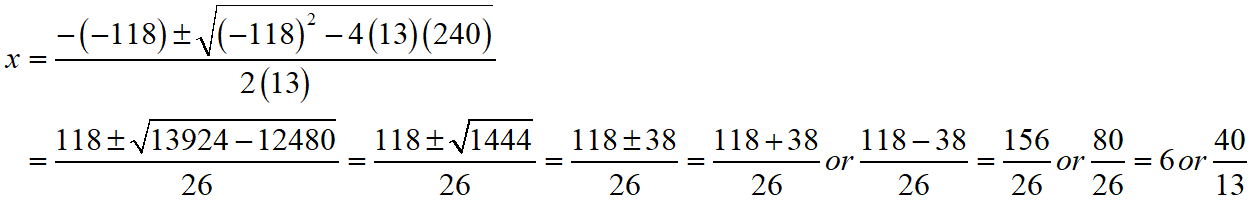Thus, the roos of the given equation are x = 6 or 40/13.

S Chand ICSE Solutions Class 10 Math Quadratic Equations Exercise 5C: Ques No 19

(x + 6)/(x + 7) – (x + 1)/(x + 2) = 1/(3x + 1)

S Chand ICSE Maths Solutions:

Given that (x + 6)/(x + 7) – (x + 1)/(x + 2) = 1/(3x + 1)

⇒ [(x + 6)(x + 2) – (x + 1)(x + 7)]/(x + 7)(x + 2) = 1/(3x + 1)

⇒ (3x + 1)(x2 + 8x + 12 – x2 – 8x – 7) = (x2 + 9x + 14)

⇒ 5(3x + 1) = (x2 + 9x + 14)

⇒ 15x + 5 = x2 + 9x + 14

⇒ x2 – 6x + 9 = 0

Then, comparing this quadratic equation with ax2 + bx + c = 0, we get a = 1, b = -6, c = 9

Putting the values of a, b and c quadratic formula x = [- b ± √(b2 – 4ac)]/2a.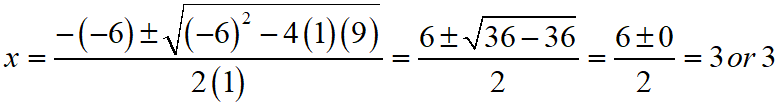Thus, the roos of the given equation are x = 3 or 3.

S Chand ICSE Solutions Class 10 Math Quadratic Equations Exercise 5C: Ques No 20

(x + 1)/(2x + 5) = (x + 3)/(3x + 4)

S Chand ICSE Maths Solutions:

Given that (x + 1)/(2x + 5) = (x + 3)/(3x + 4)

⇒ (x + 1)(3x + 4) = (x + 3)(2x + 5)

⇒ 3x2 + 7x + 4 = 2x2 + 11x + 15

⇒ x2 – 4x – 11 = 0

Then, comparing this quadratic equation with ax2 + bx + c = 0, we get a = 1, b = -4, c = -11

Putting the values of a, b and c quadratic formula x = [- b ± √(b2 – 4ac)]/2a.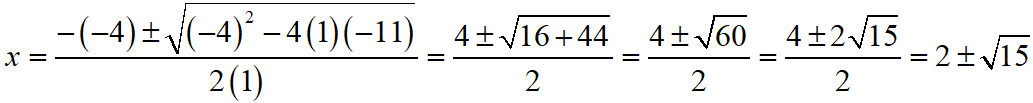Thus, the roos of the given equation are x = 2 + √15 or 2 – √15.

S Chand ICSE Solutions Class 10 Math Quadratic Equations Exercise 5C: Ques No 21(i)

Solve using quadratic formula : a2x2 – 3abx + 2b2 = 0

S Chand ICSE Maths Solutions:

Given that a2x2 – 3abx + 2b2 = 0

Then, comparing this quadratic equation with Ax2 + Bx + C = 0, we get A = a2, B = -3ab, C = 2b2

Putting the values of A, B and C quadratic formula x = [- B ± √(B2 – 4AC)]/2A.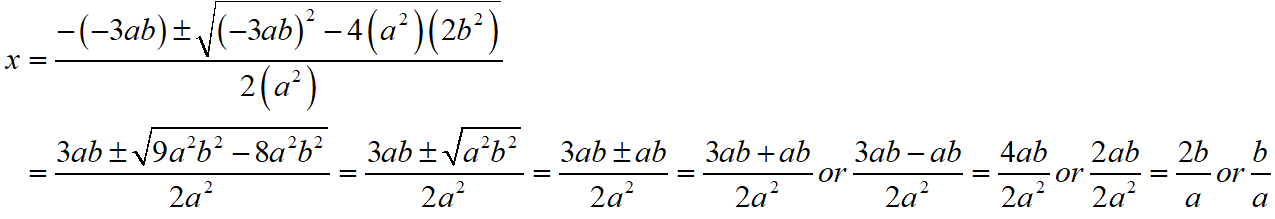Thus, the roos of the given equation are x = 2b/a or b/a.

S Chand ICSE Solutions Class 10 Math Quadratic Equations Exercise 5C: Ques No 21(ii)

Solve using quadratic formula : x2 – x – a(a + 1) = 0

S Chand ICSE Maths Solutions:

Given that x2 – x – a(a + 1) = 0

Then, comparing this quadratic equation with Ax2 + Bx + C = 0, we get A = 1, B = -1, C = -a(a + 1)

Putting the values of A, B and C quadratic formula x = [- B ± √(B2 – 4AC)]/2A.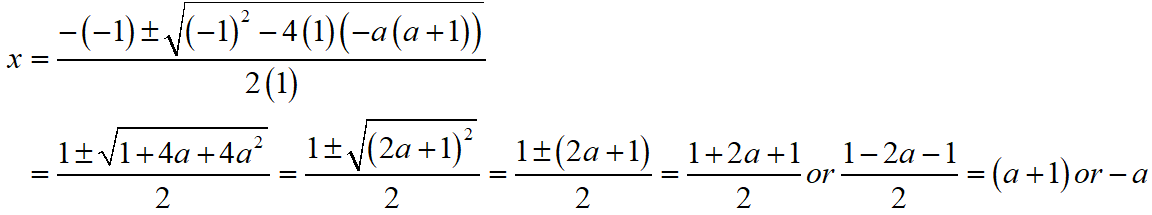Thus, the roos of the given equation are x = -a or (a + 1).

S Chand ICSE Solutions Class 10 Math Quadratic Equations Exercise 5C: Ques No 21(iii)

Solve using quadratic formula : 10x2 + 3bx + a2 – 7ax – b2 = 0

S Chand ICSE Maths Solutions:

Given that 10x2 + 3bx + a2 – 7ax – b2 = 0

10x2 + (3b – 7a)x + (a2 – b2) = 0

Then, comparing this quadratic equation with Ax2 + Bx + C = 0, we get A = 10, B = (3b – 7a), C = (a2 – b2).

Putting the values of A, B and C quadratic formula x = [- B ± √(B2 – 4AC)]/2A.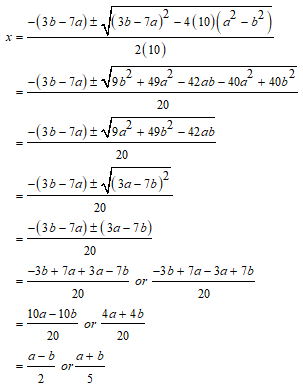error: Content is protected !!